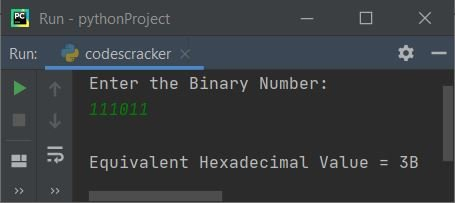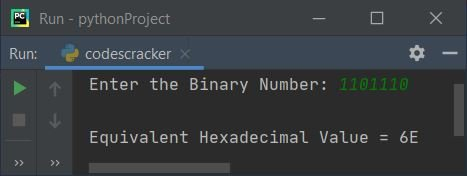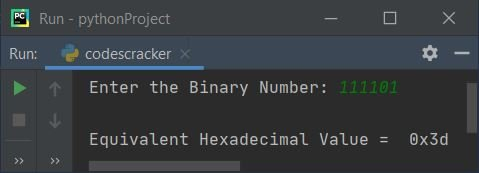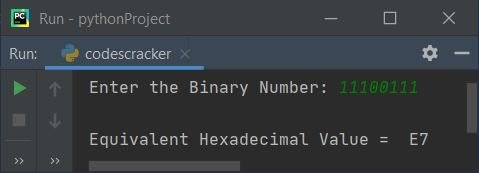# Python Program to Convert Binary to Hexadecimal

In this article, we've created some programs in Python, to convert any binary number entered by user to its equivalent hexadecimal value. Here are the list of programs:

• Binary to Hexadecimal with User-defined Code
• Binary to Hexadecimal using int() and hex() Methods

Note - Before creating these programs, if you're not aware about some steps used for the conversion, refer to Binary to Hexadecimal Conversion Steps and Formula to get every required things.

## Binary to Hexadecimal with User-defined Code

To convert binary to hexadecimal number in Python, you have to ask from user to enter a number in binary number system to convert that number into hexadecimal number system as shown in the program given here.

The question is, write a Python program to convert binary to hexadecimal using while loop. Here is its answer:

```print("Enter the Binary Number: ")
bnum = int(input())

hex = 0
mul = 1
chk = 1
i = 0
hnum = []
while bnum!=0:
rem = bnum%10
hex = hex + (rem*mul)
if chk%4==0:
if hex<10:
hex = hex+48
val = chr(hex)
hnum.insert(i, val)
else:
hex = hex+55
val = chr(hex)
hnum.insert(i, val)
mul = 1
hex = 0
chk = 1
i = i+1
else:
mul = mul*2
chk = chk+1
bnum = int(bnum/10)

if chk!=1:
hex = hex+48
val = chr(hex)
hnum.insert(i, val)
if chk==1:
i = i-1

print("\nEquivalent Hexadecimal Value = ", end="")
while i>=0:
print(end=hnum[i])
i = i-1
print()```

Here is the initial output produced by this Python program:Now supply the input say 111011 and press `ENTER` key to convert it into its equivalent hexadecimal value and print the hexadecimal value on output as shown in the snapshot given below:Note - The chr() method is used to returns equivalent character to the Unicode specified as its argument.

The insert() method is used to insert an element to the list. Therefore the following statement:

`hnum.insert(i, val)`

can be treated as:

`hnum[i] = val`

That is, the value of val variable gets initialized to ith index of hnum list.

The dry run of above program, with user input 111011 goes like:

• Initial values, bnum=111011 (entered by user), hex=0, mul=1, chk=1, i=0
• The condition (of while loop) bnum!=0 or 111011!=0 evaluates to be true, therefore program flow goes inside the loop
• Inside the loop, the first statement, that is:
`rem = bnum%10`
gets executed
• Therefore bnum%10 or 111011%10 or 1 gets initialized to rem
• hex + (rem*mul) or 0 + (1*1) or 1 gets initialized to hex
• The condition (of if) chk%4==0 or 1%4==0 evaluates to be false, therefore program flow does not goes inside this if's block, rather it goes to its else's part
• And mul*2 or 1*2 or 2 gets initialized to mul. So mul=2
• chk+1 or 1+1 or 2 gets initialized to chk. So chk=2
• And finally, int(bnum/10) or int(111011/10) or 11101 gets initialized to bnum
• The condition (of while loop) bnum!=0 again gets evaluated with new value of bnum. That is, bnum!=0 or 11101!=0 evaluates to be true again, therefore program flow goes inside the loop again. This process continues, until the condition evaluates to be false
• In this way, the binary number entered by user gets converted into its equivalent hexadecimal value

The condition:

`if chk%4==0:`

is applied to check four-four pair of binary digits to convert into its equivalent hexadecimal digit.

And the condition:

`if hex<10:`

is applied to check whether the equivalent hexadecimal digit is less than 10 or not. That is, if it is 10, 11, 12, ..., 15. Then we've to store A, B, C, ..., F in place of these.

For example, if hex digit is 12, then the condition hex<10 evaluates to be false, therefore its else's counterpart gets executed. And in place of 12 we've to store C. Therefore C gets stored through following block of code:

```hex = hex+55
val = chr(hex)
hnum.insert(i, val)```

Since hex=12, therefore hex+55 or 12+55 or 67 gets initialized to hex. And chr(hex) returns the corresponding character equals to the Unicode specified as its argument, that is the value of hex, 67. Since 67 is the ASCII value of C. Therefore val = C. And the value of val gets initialized to ith index of hnum.

#### Modified Version of Previous Program

This program is the modified version of previous one. The end= is used to skip printing of an automatic newline using print().

```print("Enter the Binary Number: ", end="")
bnum = int(input())

hex = 0
mul = 1
chk = 1
i = 0
hnum = []
while bnum!=0:
rem = bnum%10
hex = hex + (rem*mul)
if chk%4==0:
if hex<10:
hnum.insert(i, chr(hex+48))
else:
hnum.insert(i, chr(hex+55))
mul = 1
hex = 0
chk = 1
i = i+1
else:
mul = mul*2
chk = chk+1
bnum = int(bnum/10)

if chk!=1:
hnum.insert(i, chr(hex+48))
if chk==1:
i = i-1

print("\nEquivalent Hexadecimal Value = ", end="")
while i>=0:
print(end=hnum[i])
i = i-1
print()```

Here is its sample run with user input, 1101110:## Binary to Hexadecimal using int() and hex()

This program uses int() and hex() methods to do the job of converting binary to hexadecimal.

```print("Enter the Binary Number: ", end="")
bnum = input()

b = int(bnum, 2)
hdnum = hex(b)
print("\nEquivalent Hexadecimal Value = ", hdnum)```

Here is its sample run with user input 111101 as binary number:While receiving the input, we've not specified the type, therefore the entered value by user gets treated as a string type value. And using int() method with 2 as its second argument, converted the given value into an integer type value of base 2, that is binary number.

And using hex() method, the binary number gets converted into its equivalent hexadecimal value.

#### Modified Version of Previous Program

This program uses hdnum[2:] to print element(s) starting from second index. That is, the first two characters gets skipped after applying [2:]. And upper() is used to capitalize the lowercase character.

```print("Enter the Binary Number: ", end="")
bnum = input()

b = int(bnum, 2)
hdnum = hex(b)
print("\nEquivalent Hexadecimal Value = ", hdnum[2:].upper())```

Here is its sample run with user input, 11100111:#### Same Program in Other Languages

Python Online Test

« Previous Program Next Program »